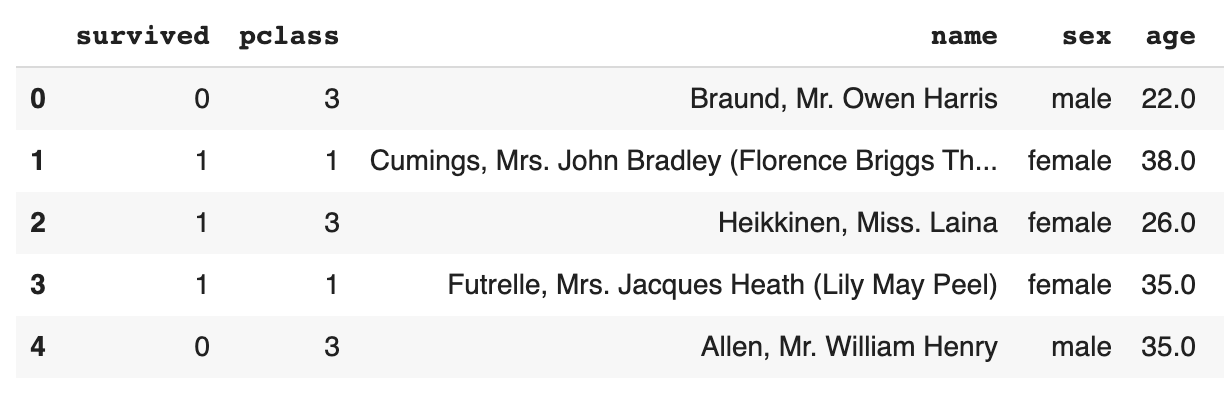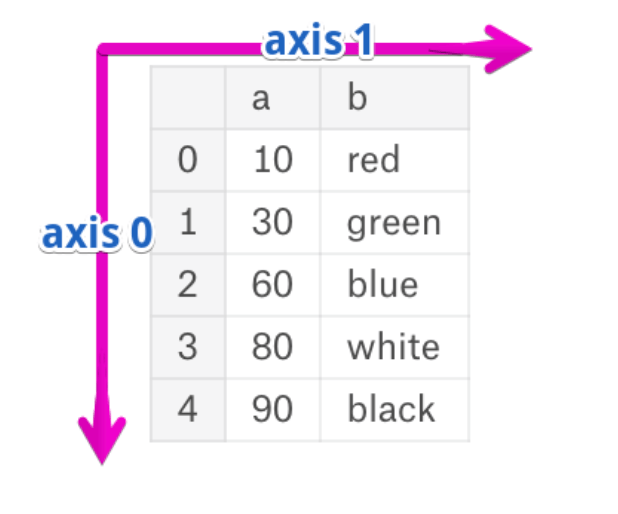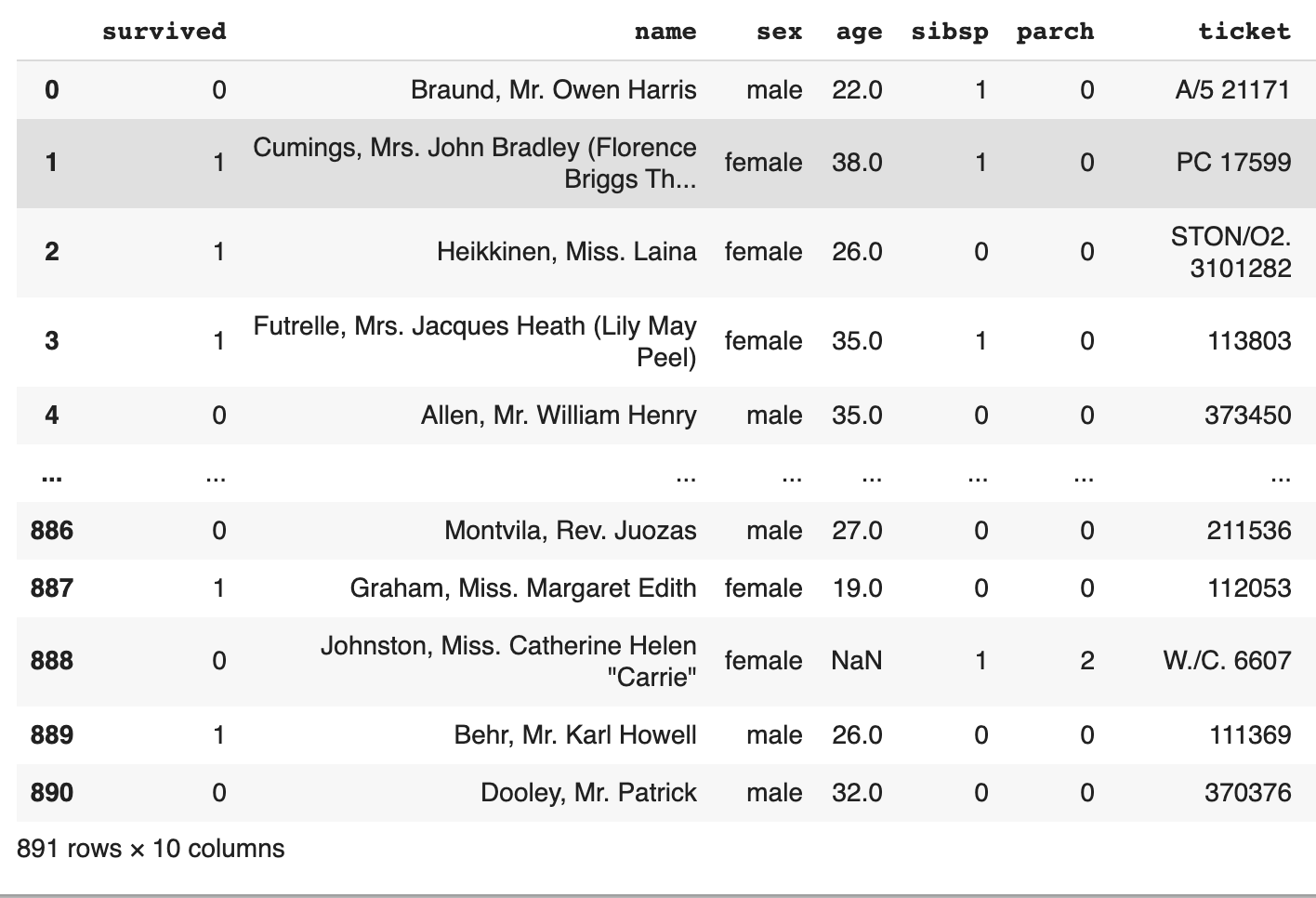# How to drop columns in a pandas dataframe

2

Pandas is a great tool for working on any machine learning or data science project. It’s a fundamental part of data wrangling. In this tutorial, we will show you how to drop a column in a pandas dataframe.

In order to drop a column in pandas, either select all the columns by using axis or select columns to drop with the drop method in the pandas dataframe.

The goals are to show both methods for dropping a column. The full code in Google Colabs is available to save or copy from directly since code can get kind of ugly in a web post.

## The first way to drop columns in a pandas dataframe is by using axis.

For the following dataframe you will see there is a column called pclass.## In order to drop pclass add the following code where “titanic” is our dataframe.

See full code.

 titanic.drop(['pclass'], axis=1)

view raw

titanicdrop1.py

hosted with ❤ by GitHub

This code drops the column pclass. But what is that axis=1 you ask?
axis=1 is the column. If it were axis=0 it would be the row.### There is another way to drop a column from a pandas dataframe, which is by using column instead of axis=1.titanic.drop(columns=['sex'])

Full code example below: